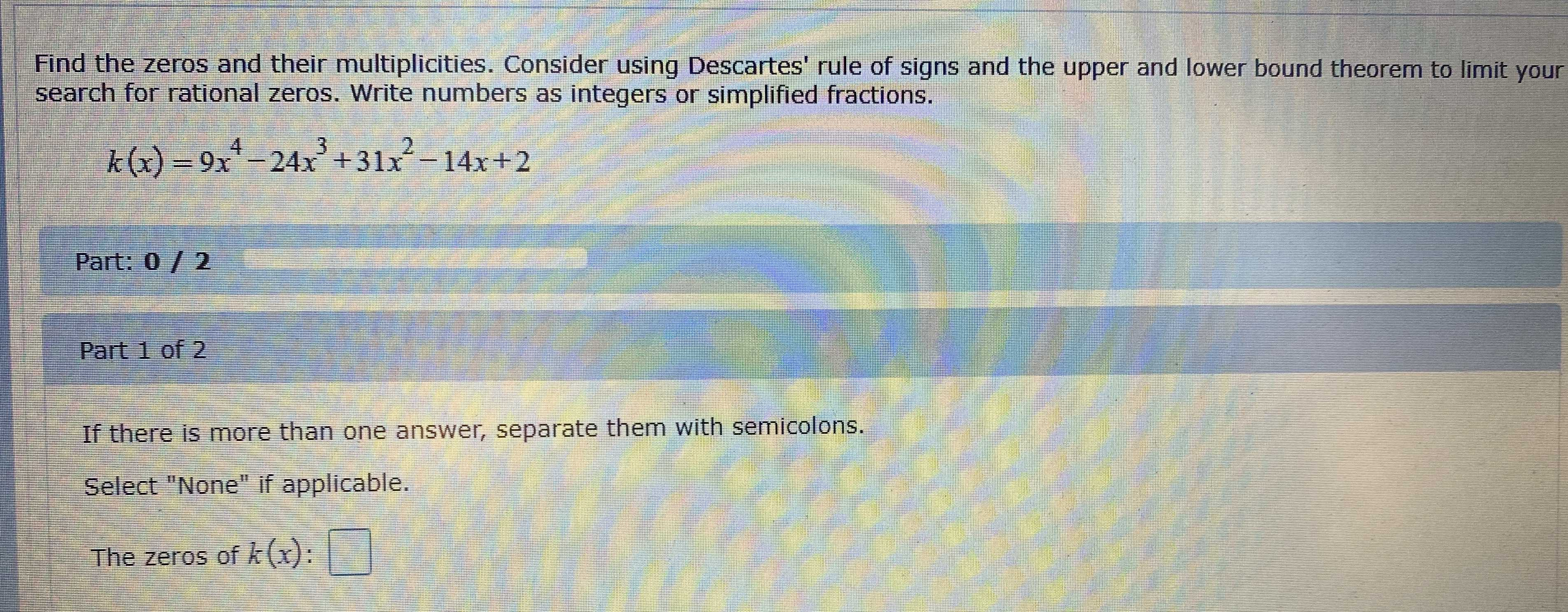### Still have math questions?

Algebra
QuestionFind the zeros and their multiplicities. Consider using Descartes' rule of signs and the upper and lower bound theorem to limit your search for rational zeros. Write numbers as integers or simplified fractions.

$$k ( x ) = 9 x ^ { 4 } - 24 x ^ { 3 } + 31 x ^ { 2 } - 14 x + 2$$

Part: $$0 / 2$$

Part $$1$$ of $$2$$

If there is more than one answer, separate them with semicolons.

Select "None" if applicable.

$$\frac{1}{3}$$